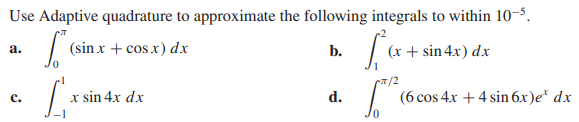# Question Use Adaptive quadrature to approximate the following integrals to within $10^{-5}$ . a. $\int_{0}^{\pi}(\sin x+\cos x) d x$ b. $\int_{1}^{2}(x+\sin 4 x) d x$ c. $\int_{-1}^{1} x \sin 4 x d x$ d. $\int_{0}^{\pi / 2}(6 \cos 4 x+4 \sin 6 x) e^{x} d x$RZ5NW0 The Asker · Other Mathematics
Use Adaptive quadrature to approximate the following integrals to within   $10^{-5}$  .
a.   $\int_{0}^{\pi}(\sin x+\cos x) d x$
b.   $\int_{1}^{2}(x+\sin 4 x) d x$
c.   $\int_{-1}^{1} x \sin 4 x d x$
d.   $\int_{0}^{\pi / 2}(6 \cos 4 x+4 \sin 6 x) e^{x} d x$Transcribed Image Text: Use Adaptive quadrature to approximate the following integrals to within $10^{-5}$ . a. $\int_{0}^{\pi}(\sin x+\cos x) d x$ b. $\int_{1}^{2}(x+\sin 4 x) d x$ c. $\int_{-1}^{1} x \sin 4 x d x$ d. $\int_{0}^{\pi / 2}(6 \cos 4 x+4 \sin 6 x) e^{x} d x$
More
Transcribed Image Text: Use Adaptive quadrature to approximate the following integrals to within $10^{-5}$ . a. $\int_{0}^{\pi}(\sin x+\cos x) d x$ b. $\int_{1}^{2}(x+\sin 4 x) d x$ c. $\int_{-1}^{1} x \sin 4 x d x$ d. $\int_{0}^{\pi / 2}(6 \cos 4 x+4 \sin 6 x) e^{x} d x$